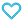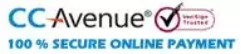# Objective Mathematics For JEE Main and Advanced

800.00

MTG’s Objective Mathematics for JEE Main & Advanced is a complete book designed for all JEE aspirants with new knowledge and advanced chapter wise techniques. The Book is divided into two sections, Class 11 and Class 12 as per the NCERT syllabus. Each chapter begins with synopsis to understand the concepts and formulae for solving the problem in a better way.

This book covers variety of questions asked in the JEE Main and Advanced examination. It covers more than 5800 objective questions of single correct option, more than one correct options, assertion and reason type, comprehension type, matrix type and integer answer type to strengthen the question solving skills of the student. This book covers ample number of illustrations and warmup session to help the aspirants for JEE preparation. The solutions are short and crisp helping students save time during exams.

It covers previous years questions of JEE from 2013 to 2019 which results in understanding the changing pattern of questions asked in the previous examinations. 6 assignments are segregated chapter wise and topic wise for self-analysis of preparation.

949 in stock

Qty:Add to WishlistContent:
• CLASS XI
• 2019 & 2018 JEE Main & Advanced
• 1. Sets, Relations and Functions
• 2. Trigonometric Functions
• 3. Principle of Mathematical Induction
• 4. Complex Numbers and Quadratic Equations
• 5. Linear Inequalities
• Assiggnment-1
• 6 Permutations and Combinations
• 7. Binomial Theorem
• 8. Sequences and Series
• 9. Limits and Derivatives
• 10. Straight Lines
• Assignment-2
• 11. Conic Sections
• 12. Introduction to Three-Dimensional Geometry
• 13. Mathematical Reasoning
• 14. Statistics and Probability
• 15. Logarithms
• Assignment-3
• CLASS XII
• 1. Relations and Functions
• 2. Inverse Trigonometric Functions
• 3. Matrices
• 4. Determinants
• Assignment-1
• 5. Continuity and Differentiability
• 6. Application of Derivatives
• 7. Integrals
• 8. Application of Integrals
• 9. Differential Equations
• Assignment-2
• 10. Vector Algebra
• 11. Three-Dimensional Geometry
• 12. Linear Programming
• 13. Probability
• Assignment-3
ISBN11 9789389167597 Dr. Ramanaiah Gundala 2019-20 1024 Class 11, Class 12 JEE Mains-Advanced Mathematics 1526gm

## Reviews

There are no reviews yet.